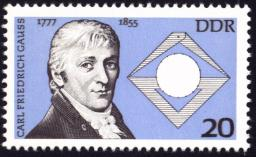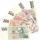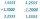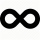# Fraction

Fraction
$\frac{0,\stackrel{‾}{38}}{0,38}$
write as fraction a/b, a, b is integers numerator/denominator.

a =  100
b =  99

### Step-by-step explanation:

$b=99=99$Did you find an error or inaccuracy? Feel free to write us. Thank you!Stella
i like this

5 years ago  1 LikeBob
good

5 years ago  1 LikeSweetmayumih1988
Thank you allTips to related online calculators
Need help to calculate sum, simplify or multiply fractions? Try our fraction calculator.

## Related math problems and questions:

• Decimal to fractionWrite decimal number 8.638333333 as a fraction A/B in the basic form. Given decimal has infinite repeating figures.
• Series and sequencesFind a fraction equivalent to the recurring decimal? 0.435643564356
• Infinite decimalImagine the infinite decimal number 0.99999999 .. ... ... ... That is a decimal and her endless series of nines. Determine how much this number is less than the number 1. Thank you in advance.
• Sum of seriesDetermine the 6-th member and the sum of a geometric series: 5-4/1+16/5-64/25+256/125-1024/625+....
• Denominator 2Denominator of a fraction is 5 and numerator is 7. Write the fraction .
• Infinite sum of areasAbove the height of the equilateral triangle ABC is constructed an equilateral triangle A1, B1, C1, of the height of the equilateral triangle built A2, B2, C2, and so on. The procedure is repeated continuously. What is the total sum of the areas of all tr
• A fractionI think of a fraction, if we increase its numerator and denominator by one, the value of the fraction increases by one tenth, what fraction do I think?
• When 5When 5 is subtracted from both my numerator and denominator I become 3/4. What fraction i am?
• Saving per centsThe first day I save 1 cent and every next day cent more. How many I saved per year (365 days)?
• Over fractionSolve equation: p over 5 equals fraction numerator 7 p over denominator 6 end fraction plus 5
• Find twoFind two consecutive natural numbers whose product is 1 larger than their sum. Searched numbers expressed by a fraction whose numerator is the difference between these numbers and the denominator is their sum.
• Fraction to decimal infiniteDetermine which digit is at 1000th place after the decimal point in the decimal expansion of the fraction 9/28.
• Guess a fractionTom was asked to guess a fraction. The sum of 1/2 the numerator and 1/3 of its denominator is 30. If Tom subtracts 36 from its denominator, the fraction becomes 1/3. What is the fraction that Tom was asked to guess? (Leave your answer in simplest form)
• Division by zeroFraction 5 by 2. if 3 is added to numerator and 2 is subtracted from the denominator then the new fraction is:
• Sum 1-6Find the sum of the geometric progression 3, 15, 75,… to six terms.
• Progression-12, 60, -300,1500 need next 2 numbers of pattern
• Probably memberLook at the series 2,6,25,96,285, ? What number should come next?